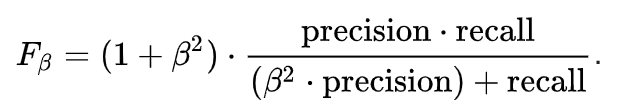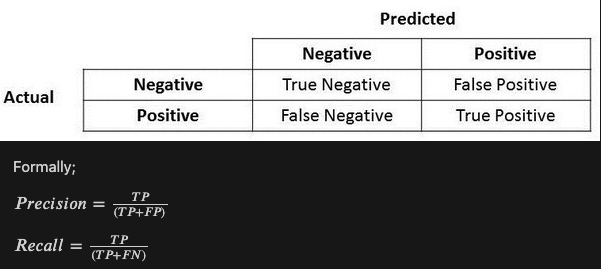# Detailed overview of F1 Score

In the last section, we discussed precision and recall for classification problems and also highlighted the importance of choosing precision/recall basis our use case. What if for a use case, we are trying to get the best precision and recall at the same time? F1-Score is the harmonic mean of precision and recall values for a classification problem. The formula for F1-Score is as follows:

Now, an obvious question that comes to mind is why are taking a harmonic mean and not an arithmetic mean. This is because HM punishes extreme values more. Let us understand this with an example. We have a binary classification model with the following results:

Precision: 0, Recall: 1

Here, if we take the arithmetic mean, we get 0.5. It is clear that the above result comes from a dumb classifier which just ignores the input and just predicts one of the classes as output. Now, if we were to take HM, we will get 0 which is accurate as this model is useless for all purposes.

This seems simple. There are situations however for which a data scientist would like to give a percentage more importance/weight to either precision or recall. Altering the above expression a bit such that we can include an adjustable parameter beta for this purpose, we get:Fbeta measures the effectiveness of a model with respect to a user who attaches β times as much importance to recall as precision.

F1 score is used as a performance metric for classification algorithms.

Firstly we need to know about the confusion matrix. Confusion matrix comes into the picture when you have already build your model.

This is what a confusion matrix looks like.
Predicted is the values that have been predicted by the model on the validation set. Actual is the ground truth available to you in the validation set.